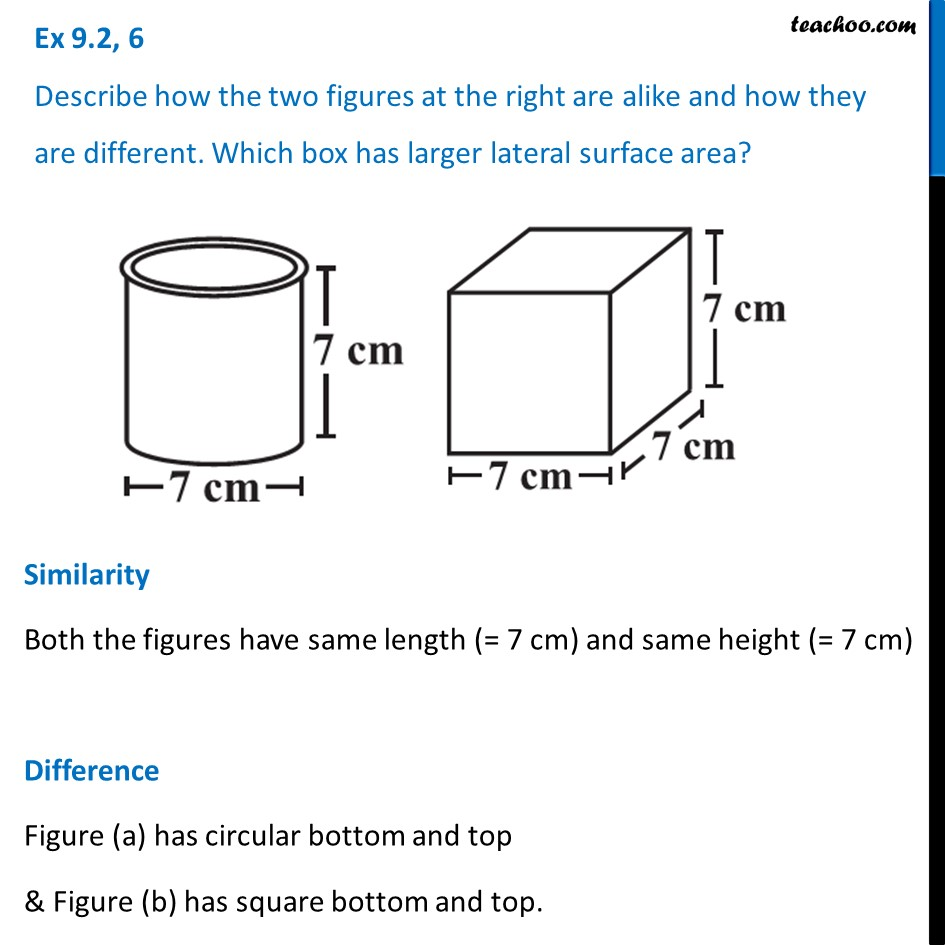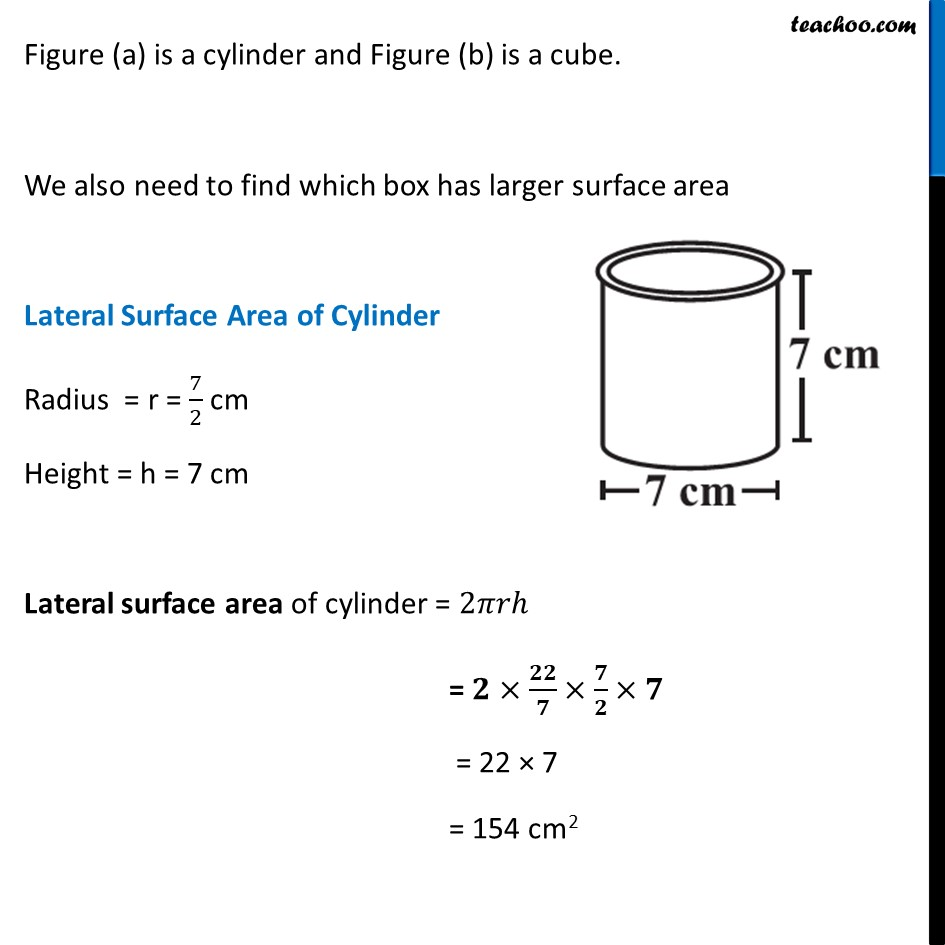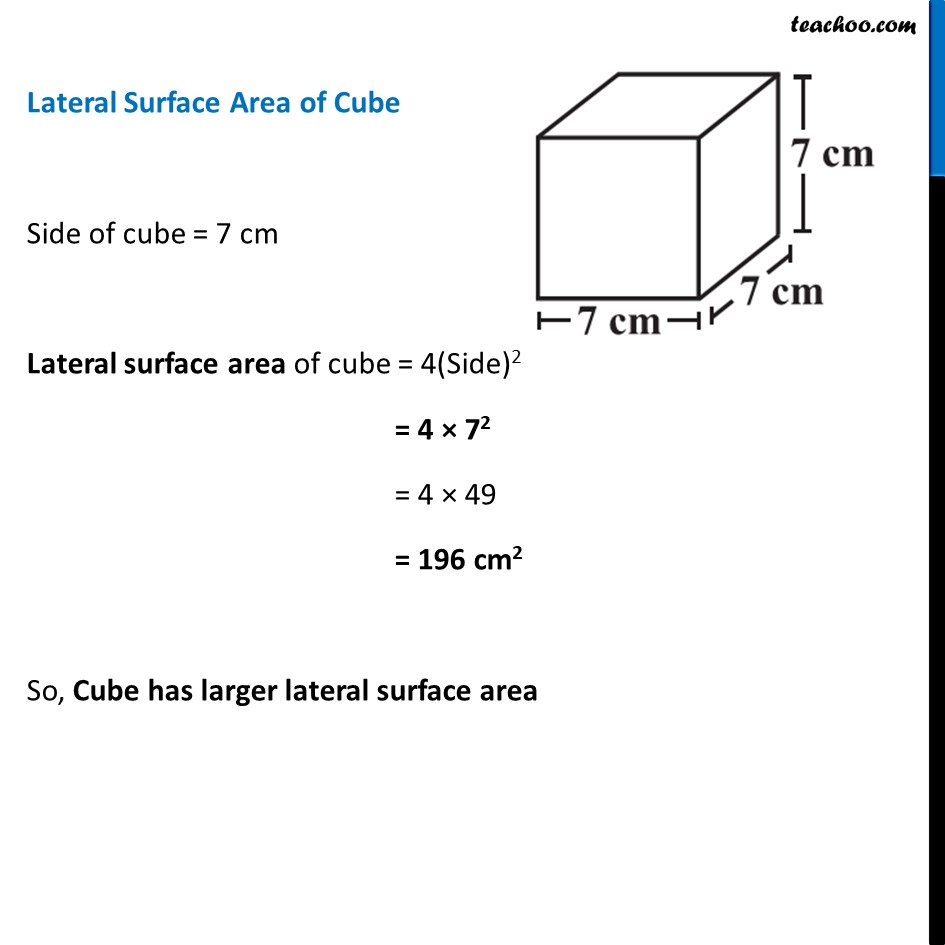Ex 9.2

Chapter 9 Class 8 Mensuration
Serial order wiseLearn in your speed, with individual attention - Teachoo Maths 1-on-1 Class

### Transcript

Ex 9.2, 6 Describe how the two figures at the right are alike and how they are different. Which box has larger lateral surface area?Similarity Both the figures have same length (= 7 cm) and same height (= 7 cm) Difference Figure (a) has circular bottom and top & Figure (b) has square bottom and top. Figure (a) is a cylinder and Figure (b) is a cube. Figure (a) is a cylinder and Figure (b) is a cube. We also need to find which box has larger surface area Lateral Surface Area of Cylinder Radius = r = 7/2 cm Height = h = 7 cm Lateral surface area of cylinder = 2𝜋𝑟ℎ = 𝟐×𝟐𝟐/𝟕×𝟕/𝟐×𝟕 = 22 × 7 = 154 cm2 Lateral Surface Area of Cube Side of cube = 7 cm Lateral surface area of cube = 4(Side)2 = 4 × 72 = 4 × 49 = 196 cm2 So, Cube has larger lateral surface area